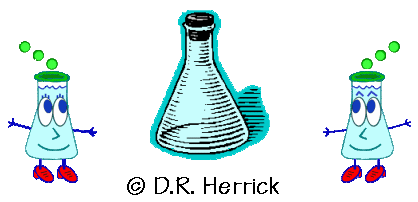Dr. Herrick's CH223 Pressure Quiz 5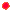More Titrations, Ionic Equilibria Copyright  D. Herrick20 Questions from past exams. Practice for speed.  Aim for 2 minutes per problem. (all concentrations in molarity units)
 Several questions involve sulfurous acid, H2SO3, which has       Ka1  =  1.4 × 10 -2  ,       Ka2  =  6.5 × 10 -8.
 1 Use appropriate combinations of the Bronsted reactions for Ka, Kb, and Kw to determine which equilibrium constant is NOT correct for the neutralization reaction (1 minute each):
 A) H2SO3 + OH-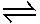HSO3- + H2O ,  K = 1.4 × 1012 B) HSO3- + OH-SO32- + H2O ,  K = 6.5 × 106 C) SO32- + 2H3O+H2SO3 + 2H2O,  K = 1.1 × 109 D) HSO3- + H3O+H2SO3 + H2O,  K = 7.1 × 101 E) SO32- + H2SO32HSO3- ,  K = 2.2 × 105 F) OH- + H3O+2H2O,  K = 1.0 × 1014 G) none of the above2 What volume of 0.10 M NaOH will titrate 50 mL of 0.1 M H2SO3 to the first equivalence point?
 A) 25 mL B) 50 C) 75 D) 100 E) 125
 3 What volume of 0.10 M NaOH will titrate 50 mL of 0.1 M H2SO3 to the second equivalence point?
 A) 25mL B) 50 C) 75 D) 100 E) 125
 4 What volume of 0.10 M NaOH will titrate 50 mL of 0.1 M H2SO3 to the second buffer point?
 A) 25mL B) 50 C) 75 D) 100 E) 125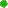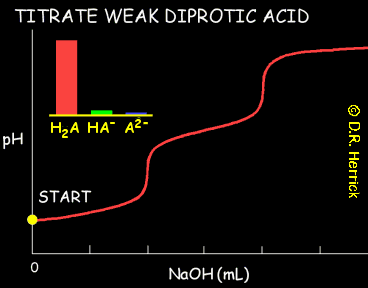5 [H+] in 0.1 M H2SO3 is: (quadratic equation is needed here due to high degree of ionization)
 A) 3.0 × 10-2 D) 3.5 × 10-2 B) 3.1 × 10-2 E) 3.7 × 10-2 C) 3.3 × 10-2 F) 3.9 × 10-2
 6 [SO32-] in 0.1 M H2SO3 is:
 A) 2.5 × 10-4 D) 9.1 × 10-9 B) 1.4 × 10-3 E) 3.9 × 10-12 C) 4.7 × 10-6 F) 6.5 × 10-8
 7 A solution prepared as 0.10 M Na2SO3 has:
 A) [ H2SO3 ]  >  [ HSO3 – ]  >  [ SO3 2 – ] B) [ H2SO3 ]  =  [ HSO3 – ]  >  [ SO3 2 – ] C) [ HSO3 – ]  >  [ H2SO3 ]  =  [ SO3 2 – ] D) [ HSO3 – ]  =  [ SO3 2 – ]  >  [ H2SO3 ] E) [ SO3 2 – ]  >  [ HSO3 – ]  >  [ H2SO3 ] F) [ SO3 2 – ]  >  [ HSO3 – ]  =  [ H2SO3 ]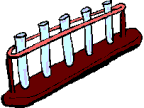8 A solution prepared as 0.10 M NaHSO3 has:
 A) [ H2SO3 ]  >  [ HSO3 – ]  >  [ SO3 2 – ] B) [ H2SO3 ]  =  [ HSO3 – ]  >  [ SO3 2 – ] C) [ HSO3 – ]  >  [ H2SO3 ]  =  [ SO3 2 – ] D) [ HSO3 – ]  =  [ SO3 2 – ]  >  [ H2SO3 ] E) [ SO3 2 – ]  >  [ HSO3 – ]  >  [ H2SO3 ] F) [ SO3 2 – ]  >  [ HSO3 – ]  =  [ H2SO3 ]
 9 A solution prepared as 0.10 M H2SO3 has:
 A) [ H2SO3 ]  >  [ HSO3 – ]  >  [ SO3 2 – ] B) [ H2SO3 ]  =  [ HSO3 – ]  >  [ SO3 2 – ] C) [ HSO3 – ]  >  [ H2SO3 ]  =  [ SO3 2 – ] D) [ HSO3 – ]  =  [ SO3 2 – ]  >  [ H2SO3 ] E) [ SO3 2 – ]  >  [ HSO3 – ]  >  [ H2SO3 ] F) [ SO3 2 – ]  >  [ HSO3 – ]  =  [ H2SO3 ]
 10 A solution prepared as 0.10 M NaHSO3 and 0.10 M Na2SO3 has:
 A) [ H2SO3 ]  >  [ HSO3 – ]  >  [ SO3 2 – ] B) [ H2SO3 ]  =  [ HSO3 – ]  >  [ SO3 2 – ] C) [ HSO3 – ]  >  [ H2SO3 ]  =  [ SO3 2 – ] D) [ HSO3 – ]  =  [ SO3 2 – ]  >  [ H2SO3 ] E) [ SO3 2 – ]  >  [ HSO3 – ]  >  [ H2SO3 ] F) [ SO3 2 – ]  >  [ HSO3 – ]  =  [ H2SO3 ]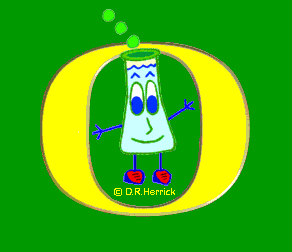11 A solution prepared as 0.10 M NaHSO3 and 0.10 M H2SO3 has:
 A) [ H2SO3 ]  >  [ HSO3 – ]  >  [ SO3 2 – ] B) [ H2SO3 ]  =  [ HSO3 – ]  >  [ SO3 2 – ] C) [ HSO3 – ]  >  [ H2SO3 ]  =  [ SO3 2 – ] D) [ HSO3 – ]  =  [ SO3 2 – ]  >  [ H2SO3 ] E) [ SO3 2 – ]  >  [ HSO3 – ]  >  [ H2SO3 ] F) [ SO3 2 – ]  >  [ HSO3 – ]  =  [ H2SO3 ]
 12 The pH after mixing 0.20 mol of H2SO3 and 0.30 mol of NaOH in 1.0 L of water is:
 A) 7.2 B) 8.9 C) 2.1 D) 5.1 E) 9.5
 13 At pH = 7.00 in a titration of H2SO3 the solution is expected to have
 A) [ H2SO3 ]  >  [ HSO3 – ]  >  [ SO3 2 – ] B) [ H2SO3 ]  >  [ SO3 2 – ]  >  [ HSO3 – ] C) [ HSO3 – ]  >  [ H2SO3 ]  >  [ SO3 2 – ] D) [ HSO3 – ]  >  [ SO3 2 – ]  >  [ H2SO3 ] E) [ SO3 2 – ]  >  [ HSO3 – ]  >  [ H2SO3 ] F) [ SO3 2 – ]  >  [ H2SO3 ]  >  [ HSO3 – ]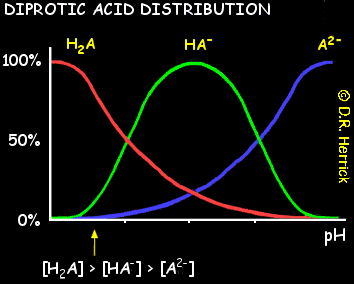14 When the pH of a solution containing H2SO3 is adjusted to pH = 7.00 the ratio [SO32-] / [HSO3- ] is
 A) 0.98 B) 0.88 C) 0.74 D) 0.65 E) 0.57
 15 When 40 mL of 0.200 M H2SO3 is titrated with 0.125 M NaOH the pH at the first equivalence point is:
 A) 9.04 D) 7.19 B) 3.64 E) 6.33 C) 1.85 F) 4.52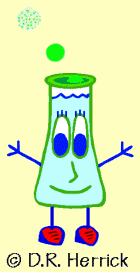16 The pH after mixing 0.30 mol of H2SO3 and 0.15 mol of NaOH in 1.0 L of water is:
 A) 5.7 B) 4.3 C) 8.6 D) 3.0 E) 1.9
 17 The molar solubility of lead iodide (PbI2 , Ksp = 6.5 × 10 -9 ) in water is:
 A) 1.9 × 10-3 D) 6.5 × 10-9 B) 1.2 × 10-3 E) 1.6 × 10-7 C) 8.3 × 10-4 F) 4.0 × 10-5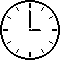18 The molar solubility of lead iodide (PbI2 , Ksp = 6.5 × 10 -9 ) in a 0.15 M solution of potassium iodide KI is:
 A) 1.9 × 10-6 D) 6.5 × 10-9 B) 1.2 × 10-4 E) 1.6 × 10-7 C) 8.1 × 10-5 F) 2.9 × 10-7
 19 What’s the molar solubility of Ca(OH)2 (Ksp = 6.5 ×10-6) in a 0.25M solution of KOH?
 A) 2.6 ×10-5 D) 5.3 ×10-4 B) 2.2 ×10-4 E) 1.0 ×10-4 C) 6.5 ×10-4 F) 4.1 ×10-7
 20 When NaOH is added slowly to a solution in which [Zn2+] = 1.0 × 10-6 the precipitate Zn(OH)2 will begin to form according to Zn(OH)2 (s)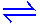Zn2+(aq) + 2 OH- (aq),  Ksp = 1.8 × 10-14 when the pH of the solution is:
 A) 10.1 B) 8.6 C) 7.2 D) 9.0 E) 12.3